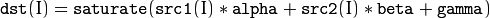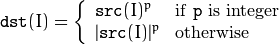# Per-element Operations¶

Computes a matrix-matrix or matrix-scalar sum.

C++: void gpu::add(const GpuMat& a, const GpuMat& b, GpuMat& c, const GpuMat& mask=GpuMat(), int dtype=-1, Stream& stream=Stream::Null() )
C++: void gpu::add(const GpuMat& a, const Scalar& sc, GpuMat& c, const GpuMat& mask=GpuMat(), int dtype=-1, Stream& stream=Stream::Null() )
Parameters: a – First source matrix. b – Second source matrix to be added to a . Matrix should have the same size and type as a . sc – A scalar to be added to a . c – Destination matrix that has the same size and number of channels as the input array(s). The depth is defined by dtype or a depth. mask – Optional operation mask, 8-bit single channel array, that specifies elements of the destination array to be changed. dtype – Optional depth of the output array. stream – Stream for the asynchronous version.

## gpu::subtract¶

Computes a matrix-matrix or matrix-scalar difference.

C++: void gpu::subtract(const GpuMat& a, const GpuMat& b, GpuMat& c, const GpuMat& mask=GpuMat(), int dtype=-1, Stream& stream=Stream::Null() )
C++: void gpu::subtract(const GpuMat& a, const Scalar& sc, GpuMat& c, const GpuMat& mask=GpuMat(), int dtype=-1, Stream& stream=Stream::Null() )
Parameters: a – First source matrix. b – Second source matrix to be added to a . Matrix should have the same size and type as a . sc – A scalar to be added to a . c – Destination matrix that has the same size and number of channels as the input array(s). The depth is defined by dtype or a depth. mask – Optional operation mask, 8-bit single channel array, that specifies elements of the destination array to be changed. dtype – Optional depth of the output array. stream – Stream for the asynchronous version.

subtract()

## gpu::multiply¶

Computes a matrix-matrix or matrix-scalar per-element product.

C++: void gpu::multiply(const GpuMat& a, const GpuMat& b, GpuMat& c, double scale=1, int dtype=-1, Stream& stream=Stream::Null() )
C++: void gpu::multiply(const GpuMat& a, const Scalar& sc, GpuMat& c, double scale=1, int dtype=-1, Stream& stream=Stream::Null() )
Parameters: a – First source matrix. b – Second source matrix to be multiplied by a elements. sc – A scalar to be multiplied by a elements. c – Destination matrix that has the same size and number of channels as the input array(s). The depth is defined by dtype or a depth. scale – Optional scale factor. dtype – Optional depth of the output array. stream – Stream for the asynchronous version.

multiply()

## gpu::divide¶

Computes a matrix-matrix or matrix-scalar division.

C++: void gpu::divide(const GpuMat& a, const GpuMat& b, GpuMat& c, double scale=1, int dtype=-1, Stream& stream=Stream::Null() )
C++: void gpu::divide(const GpuMat& a, const Scalar& sc, GpuMat& c, double scale=1, int dtype=-1, Stream& stream=Stream::Null())
C++: void gpu::divide(double scale, const GpuMat& b, GpuMat& c, int dtype=-1, Stream& stream=Stream::Null() )
Parameters: a – First source matrix or a scalar. b – Second source matrix. The a elements are divided by it. sc – A scalar to be divided by the elements of a matrix. c – Destination matrix that has the same size and number of channels as the input array(s). The depth is defined by dtype or a depth. scale – Optional scale factor. dtype – Optional depth of the output array. stream – Stream for the asynchronous version.

This function, in contrast to divide(), uses a round-down rounding mode.

divide()

Computes the weighted sum of two arrays.

C++: void gpu::addWeighted(const GpuMat& src1, double alpha, const GpuMat& src2, double beta, double gamma, GpuMat& dst, int dtype=-1, Stream& stream=Stream::Null())
Parameters: src1 – First source array. alpha – Weight for the first array elements. src2 – Second source array of the same size and channel number as src1 . beta – Weight for the second array elements. dst – Destination array that has the same size and number of channels as the input arrays. gamma – Scalar added to each sum. dtype – Optional depth of the destination array. When both input arrays have the same depth, dtype can be set to -1, which will be equivalent to src1.depth(). stream – Stream for the asynchronous version.

The function addWeighted calculates the weighted sum of two arrays as follows:where I is a multi-dimensional index of array elements. In case of multi-channel arrays, each channel is processed independently.

## gpu::abs¶

Computes an absolute value of each matrix element.

C++: void gpu::abs(const GpuMat& src, GpuMat& dst, Stream& stream=Stream::Null())
Parameters: src – Source matrix. Supports CV_16S and CV_32F depth. dst – Destination matrix with the same size and type as src . stream – Stream for the asynchronous version.

abs()

## gpu::sqr¶

Computes a square value of each matrix element.

C++: void gpu::sqr(const GpuMat& src, GpuMat& dst, Stream& stream=Stream::Null())
Parameters: src – Source matrix. Supports CV_8U , CV_16U , CV_16S and CV_32F depth. dst – Destination matrix with the same size and type as src . stream – Stream for the asynchronous version.

## gpu::sqrt¶

Computes a square root of each matrix element.

C++: void gpu::sqrt(const GpuMat& src, GpuMat& dst, Stream& stream=Stream::Null())
Parameters: src – Source matrix. Supports CV_8U , CV_16U , CV_16S and CV_32F depth. dst – Destination matrix with the same size and type as src . stream – Stream for the asynchronous version.

sqrt()

## gpu::exp¶

Computes an exponent of each matrix element.

C++: void gpu::exp(const GpuMat& a, GpuMat& b, Stream& stream=Stream::Null() )
Parameters: a – Source matrix. Supports CV_8U , CV_16U , CV_16S and CV_32F depth. b – Destination matrix with the same size and type as a . stream – Stream for the asynchronous version.

exp()

## gpu::log¶

Computes a natural logarithm of absolute value of each matrix element.

C++: void gpu::log(const GpuMat& a, GpuMat& b, Stream& stream=Stream::Null() )
Parameters: a – Source matrix. Supports CV_8U , CV_16U , CV_16S and CV_32F depth. b – Destination matrix with the same size and type as a . stream – Stream for the asynchronous version.

log()

## gpu::pow¶

Raises every matrix element to a power.

C++: void gpu::pow(const GpuMat& src, double power, GpuMat& dst, Stream& stream=Stream::Null())
Parameters: src – Source matrix. Supports all type, except CV_64F depth. power – Exponent of power. dst – Destination matrix with the same size and type as src . stream – Stream for the asynchronous version.

The function pow raises every element of the input matrix to p :pow()

## gpu::absdiff¶

Computes per-element absolute difference of two matrices (or of a matrix and scalar).

C++: void gpu::absdiff(const GpuMat& a, const GpuMat& b, GpuMat& c, Stream& stream=Stream::Null() )
C++: void gpu::absdiff(const GpuMat& a, const Scalar& s, GpuMat& c, Stream& stream=Stream::Null() )
Parameters: a – First source matrix. b – Second source matrix to be added to a . s – A scalar to be added to a . c – Destination matrix with the same size and type as a . stream – Stream for the asynchronous version.

absdiff()

## gpu::compare¶

Compares elements of two matrices.

C++: void gpu::compare(const GpuMat& a, const GpuMat& b, GpuMat& c, int cmpop, Stream& stream=Stream::Null() )
Parameters: a – First source matrix. b – Second source matrix with the same size and type as a . c – Destination matrix with the same size as a and the CV_8UC1 type. cmpop – Flag specifying the relation between the elements to be checked: CMP_EQ: a(.) == b(.) CMP_GT: a(.) < b(.) CMP_GE: a(.) <= b(.) CMP_LT: a(.) < b(.) CMP_LE: a(.) <= b(.) CMP_NE: a(.) != b(.) stream – Stream for the asynchronous version.

compare()

## gpu::bitwise_not¶

Performs a per-element bitwise inversion.

C++: void gpu::bitwise_not(const GpuMat& src, GpuMat& dst, const GpuMat& mask=GpuMat(), Stream& stream=Stream::Null())
Parameters: src – Source matrix. dst – Destination matrix with the same size and type as src . mask – Optional operation mask. 8-bit single channel image. stream – Stream for the asynchronous version.

## gpu::bitwise_or¶

Performs a per-element bitwise disjunction of two matrices or of matrix and scalar.

C++: void gpu::bitwise_or(const GpuMat& src1, const GpuMat& src2, GpuMat& dst, const GpuMat& mask=GpuMat(), Stream& stream=Stream::Null())
C++: void gpu::bitwise_or(const GpuMat& src1, const Scalar& sc, GpuMat& dst, Stream& stream=Stream::Null())
Parameters: src1 – First source matrix. src2 – Second source matrix with the same size and type as src1 . dst – Destination matrix with the same size and type as src1 . mask – Optional operation mask. 8-bit single channel image. stream – Stream for the asynchronous version.

## gpu::bitwise_and¶

Performs a per-element bitwise conjunction of two matrices or of matrix and scalar.

C++: void gpu::bitwise_and(const GpuMat& src1, const GpuMat& src2, GpuMat& dst, const GpuMat& mask=GpuMat(), Stream& stream=Stream::Null())
C++: void gpu::bitwise_and(const GpuMat& src1, const Scalar& sc, GpuMat& dst, Stream& stream=Stream::Null())
Parameters: src1 – First source matrix. src2 – Second source matrix with the same size and type as src1 . dst – Destination matrix with the same size and type as src1 . mask – Optional operation mask. 8-bit single channel image. stream – Stream for the asynchronous version.

## gpu::bitwise_xor¶

Performs a per-element bitwise exclusive or operation of two matrices of matrix and scalar.

C++: void gpu::bitwise_xor(const GpuMat& src1, const GpuMat& src2, GpuMat& dst, const GpuMat& mask=GpuMat(), Stream& stream=Stream::Null())
C++: void gpu::bitwise_xor(const GpuMat& src1, const Scalar& sc, GpuMat& dst, Stream& stream=Stream::Null())
Parameters: src1 – First source matrix. src2 – Second source matrix with the same size and type as src1 . dst – Destination matrix with the same size and type as src1 . mask – Optional operation mask. 8-bit single channel image. stream – Stream for the asynchronous version.

## gpu::rshift¶

Performs pixel by pixel right shift of an image by a constant value.

C++: void gpu::rshift(const GpuMat& src, Scalar_<int> sc, GpuMat& dst, Stream& stream=Stream::Null() )
Parameters: src – Source matrix. Supports 1, 3 and 4 channels images with integers elements. sc – Constant values, one per channel. dst – Destination matrix with the same size and type as src . stream – Stream for the asynchronous version.

## gpu::lshift¶

Performs pixel by pixel right left of an image by a constant value.

C++: void gpu::lshift(const GpuMat& src, Scalar_<int> sc, GpuMat& dst, Stream& stream=Stream::Null() )
Parameters: src – Source matrix. Supports 1, 3 and 4 channels images with CV_8U , CV_16U or CV_32S depth. sc – Constant values, one per channel. dst – Destination matrix with the same size and type as src . stream – Stream for the asynchronous version.

## gpu::min¶

Computes the per-element minimum of two matrices (or a matrix and a scalar).

C++: void gpu::min(const GpuMat& src1, const GpuMat& src2, GpuMat& dst, Stream& stream=Stream::Null())
C++: void gpu::min(const GpuMat& src1, double src2, GpuMat& dst, Stream& stream=Stream::Null())
Parameters: src1 – First source matrix. src2 – Second source matrix or a scalar to compare src1 elements with. dst – Destination matrix with the same size and type as src1 . stream – Stream for the asynchronous version.

min()

## gpu::max¶

Computes the per-element maximum of two matrices (or a matrix and a scalar).

C++: void gpu::max(const GpuMat& src1, const GpuMat& src2, GpuMat& dst, Stream& stream=Stream::Null())
C++: void gpu::max(const GpuMat& src1, double src2, GpuMat& dst, Stream& stream=Stream::Null())
Parameters: src1 – First source matrix. src2 – Second source matrix or a scalar to compare src1 elements with. dst – Destination matrix with the same size and type as src1 . stream – Stream for the asynchronous version.

max()